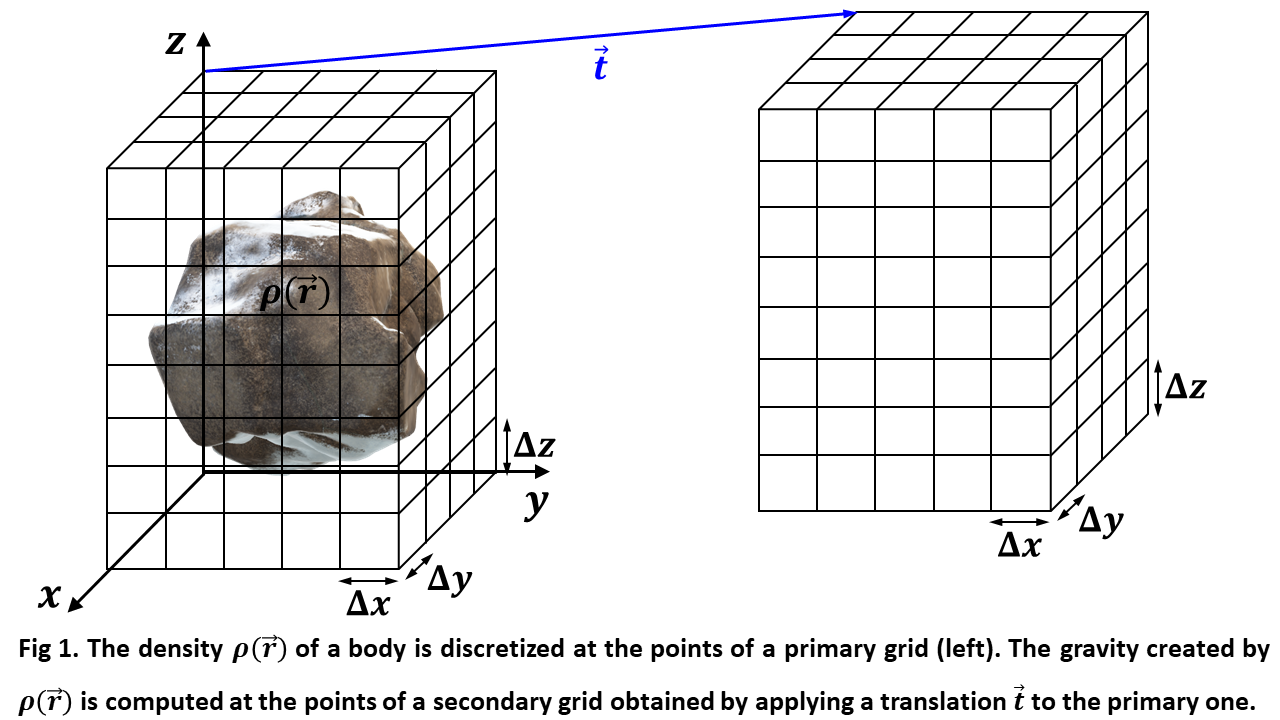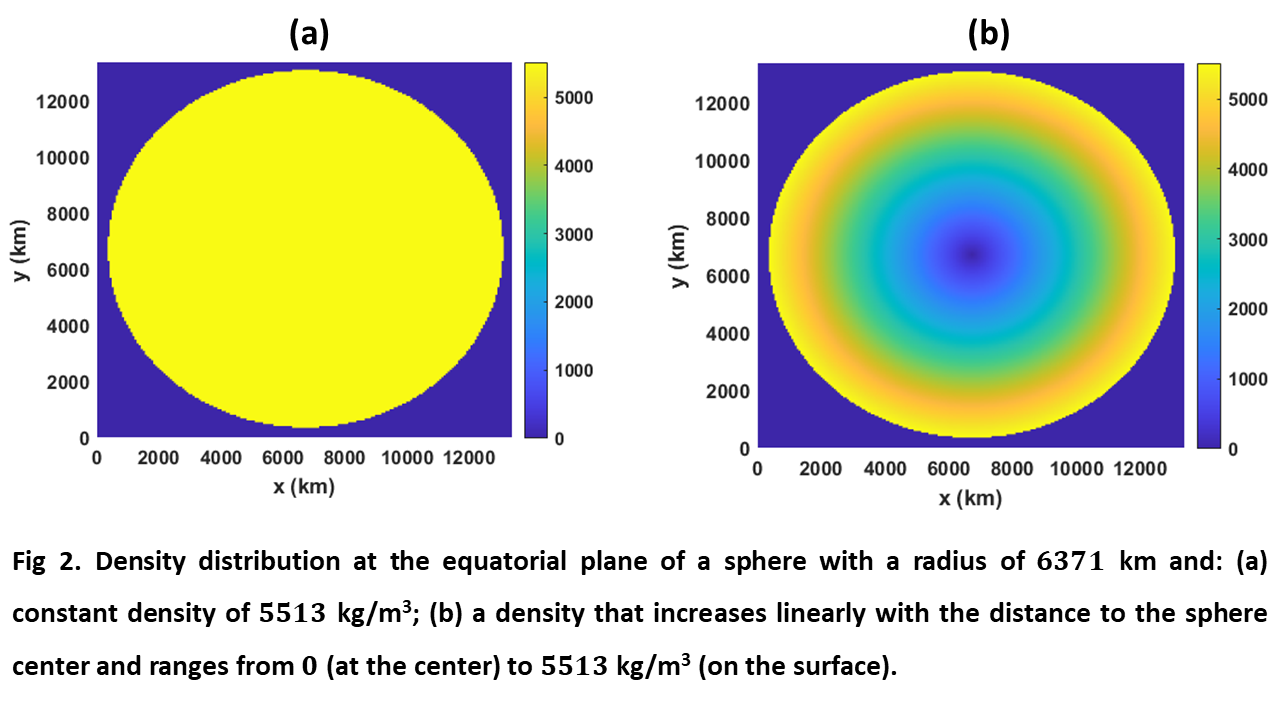Europlanet Science Congress 2021
Virtual meeting
13 September – 24 September 2021
EPSC Abstracts
Vol. 15, EPSC2021-674, 2021
https://doi.org/10.5194/epsc2021-674
Europlanet Science Congress 2021

# FFT based algorithm for efficient gravity field computations in the frame of the NEO-MAPP project

Manuel Perez-Molina et al.

Introduction

Determining the detailed gravitational field of a body is a way to get information about the internal structure of irregular shaped and non-homogenous small solar system objects, like asteroids. In the frame of the H2020 NEO-MAPP project, we are studying a solution to this problem so that suitable instrumentation can be designed onboard spacecraft visiting small bodies. Our reference asteroid is the Near-Earth Asteroid (65803) Didymos binary system, the target of the Hera mission by the European Space Agency  to be launched in late 2024.

The method proposed here is an extremely fast way to find the gravitational field in all regions of space about any body with particular attention on Didymos. This has the advantage of calculating -in a reasonable time- the gravitational fields corresponding to a large number of extremely different internal structures. Therefore, on the one hand we will be able to test the suitable resolution needed for accelerometers onboard Hera’s Juventas cubesat . On the other hand, once the Hera spacecraft will be at Didymos, measurements may be compared with a comprehensive library of potential gravitational fields. In that way, we may find what synthetic internal structure best matches spacecraft measurements.

Methodology

We propose a Fast Fourier Transform (FFT)  based algorithm for efficient computation of the gravity field created by a body with any arbitrary mass distribution defined by a mass density ρ(r) discretized with a suitable resolution. Our algorithm starts by considering a primary three-dimensional cartesian grid that contains the considered mass distribution and has uniform point spacing Δx, Δy and Δz in the directions parallel to the x, y and z axes, as shown in Figure 1. The density ρ(r) is then discretized at each primary grid points, thus characterizing the mass distribution of the body, which creates the gravity field. Next, our algorithm considers a secondary three-dimensional cartesian grid that applies an arbitrary translation to the primary one and represents the space region where the gravity field will be computed. Once the primary and secondary grids have been defined, our algorithm computes efficiently the components of the gravity vector created by the body within the primary grid at all the secondary grid points. From the computed gravity values at such points, a suitable interpolation allows extending the calculations to any point inside the region within the secondary grid.Our algorithm has been applied to bodies having regular and irregular shapes as well as uniform and non-uniform densities, and its high accuracy has been tested by comparison with other exact analytical or numerical solutions. This method is accurate for any relative position of the secondary grid concerning the primary one, including the case in which both grids coincide. Nonetheless, exceptionally high accuracy is observed when the regions within the primary and secondary grids do not overlap. Using 16 GB of RAM memory in different PCs, we achieve primary and secondary grids of up to 241 points at each axis and computation times that do not exceed one minute for each secondary grid.

Results

To test the accuracy of our method, we first compare its outcome with the analytical gravity fields of a 6371 km radius sphere in two cases: a uniform density of 5513 kg/m3 and a density that increases linearly with distance to the center up to 5513 kg/m3. Figures 2 (a) and (b) depict the density distributions of the considered spheres at their equatorial plane.Figure 3 (a) shows the gravity field modulus g computed with our method at the equatorial plane of the sphere considered in figure 2 (a) considering overlapped primary and secondary grids with 201 points in each axis. The results were obtained in 40 seconds and the deviation between the computed and exact values normalized to the maximum exact value were below 6.72·10-3. Figure 3 (b) shows the plot of such normalized deviation at the equatorial plane.